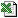Business Functions Library for Excel

Note: In order to use the spreadsheet examples, you need to download Business Functions
OptionBS (ValuationDate, OptionExpiry, RiskFreeRateAER, CostOfCarryAER, StockPrice, StrikePrice, Volatility, [OptionType], [OptionOutput], [DayCount], [Periods])
Option Value (Black/Scholes)
Calculates the price (or value) of an option using the Black/Scholes method
###### Key Points

Cost Of Carry

• If the Cost of Carry rate is the same as the Interest Rate, the formula used is the 1973 Black and Scholes model.
• If the Cost of Carry Rate is zero, the formula used is the 1976 Black futures option model.
• If the Cost of Carry Rate is equal to (Interest Rate - Dividend Yield), the model used is the 1973 Merton stock option model with dividend yield. But note that this assumes continuous dividends: if the dividends are irregular, you need to PV-adjust the price.
• If the Cost of Carry Rate is equal to the Interest Rate (risk free rate) less the Interest rate in the foreign country, this gives the 1983 Garman and Kohlhagen currency option model.

###### Key Points

Simple and Continuous Rates

• The basic Black/Scholes formula uses a continuous interest rate ie a rate that can be assumed to compound effectively daily. This function uses a Annual Effective (annually compounded) Interest Rate (AER) for both the Interest Rate and the Cost of Carry Rate because that"s a more familiar concept to many people. The function converts to a continous rate internally and if you run the Trace Facility you can see what continuous rates it has calculated..
• If your rates are in something other than AER, you can convert them with functions such as SimpleToAER and SimpleToAERX.

###### Key Points

Generalised Form of Black Scholes

• This function is the generalised Black-Scholes model, which has a Cost of Carry Rate included.
• The most important thing to remeber is that if you want basic Black/Scholes, then make the Cost of Carry Rate equal to the interest rate.

###### Key Points

DayCount and Periods

• These variables are used to establish the length of time between the Option Expiry and Valuation Dates. The function internally uses the DiffY function.
• These variables are not used to change the interest rates in any way - that is unnecessary because both rates are on a common basis (AER) and are easily converted internally to continuous rates (using AERToCont).

###### Tip

To help obtain a value for volatility, you can use the HistoricVol function.

###### ExampleOptionBS.xls

Business Functions Ltd, London, UK Website Design: Webpure